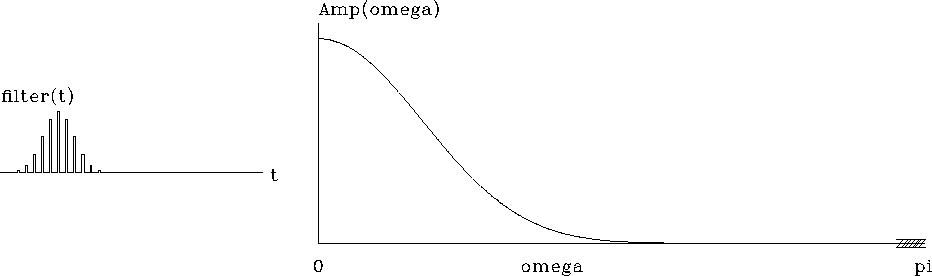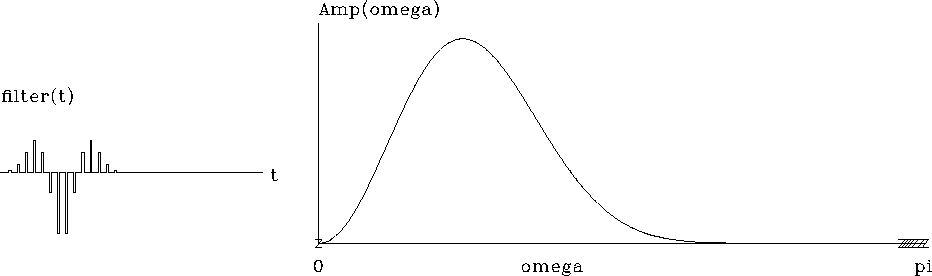Next: Complex roots Up: FOURIER AND Z-TRANSFORM Previous: Differentiator

## Gaussian examples

The filter (1+Z)/2 is a running average of two adjacent time points. Applying this filter N times yields the filter (1+Z)N/2N. The coefficients of the filter (1+Z)N are generally known as Pascal's triangle. For large N the coefficients tend to a mathematical limit known as a Gaussian function,, whereand t0 are constants that we will determine in chapter. We will not prove it here, but this Gaussian-shaped signal has a Fourier transform that also has a Gaussian shape,.The Gaussian shape is often called a bell shape.'' Figure 8 shows an example for.Note that, except for the rounded ends, the bell shape seems a good fit to a triangle function.gauss
Figure 8
A Gaussian approximated by many powers of (1+Z).

Curiously, the filter (.75+.25Z)N also tends to the same Gaussian but with a different t0. A mathematical theorem (discussed in chapter) says that almost any polynomial raised to the N-th power yields a Gaussian.

In seismology we generally fail to observe the zero frequency. Thus the idealized seismic pulse cannot be a Gaussian. An analytic waveform of longstanding popularity in seismology is the second derivative of a Gaussian, also known as a Ricker wavelet.''

Starting from the Gaussian and putting two more zeros at the origin with (1-Z)2=1-2Z+Z2 produces this old, favorite wavelet, shown in Figure 9.ricker
Figure 9
Ricker wavelet.Next: Complex roots Up: FOURIER AND Z-TRANSFORM Previous: Differentiator
Stanford Exploration Project
10/21/1998